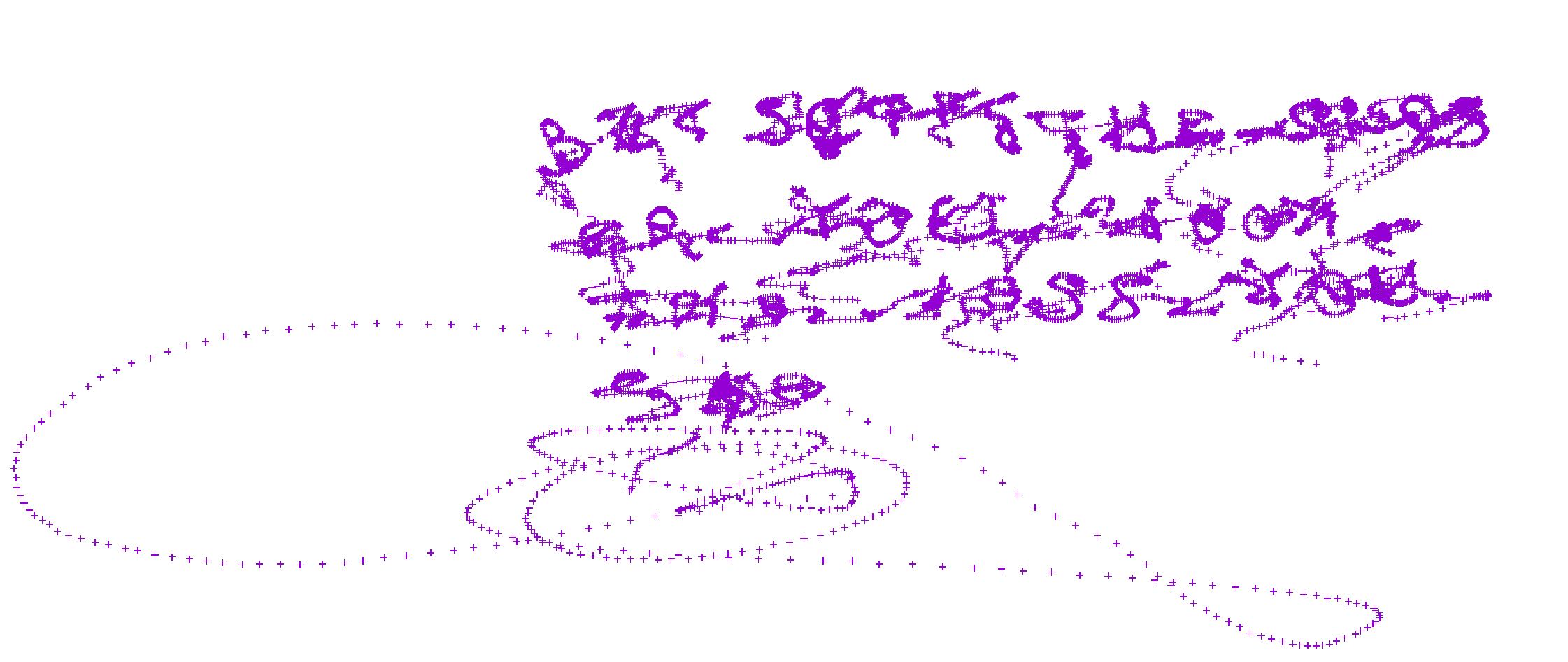# BITSCTF – Tom and Jerry (50 points)

I have a little time to join on BITSCTF with my team defconUA and want to put some writeup on one of the task i was working. They give us a pcapng named ‘Cat.pcapng’. Ok, challenge name is «Tom and Jerry» and all the things we see inside pcap is related with input devices. First thing we must check is what kind of device had been recorded on the pcap.

Seems we have a Wacom tablet with vendor and product description.

idVendor: Wacom Co., Ltd (0x056a)
idProduct: CTL-460 [Bamboo Pen (S)] (0x00d4)

From here we need what are those captured data bits and what’s the meaning of. Mainly we have packets of 73 and 64 bytes length. 64 bytes ones  are just confirmation of previous operation, so we can filter becuase there are nothing interesting there. But first, will apply as column «Leftover Captured Data» and see on the main packet window.

Now filter all non interesting packets commented previously. This can be done with simply line on wireshark.

`((usb.transfer_type == 0x01) && (frame.len == 73))`

We can ‘save as’ Cat_filtered.pcapng and work with tshark from here. But the important thing is understand how are involved those hex-bytes of captured data. Thanks to the help of teammate he points me how it works.  Let’s see.

```Example:
02:f0:50:1d:72:1a:00:00:12
Bytes:
50:1d: -- X
72:1a: -- Y
00:00: -- Pressure
12 -- Suffix```

Things come more clear now. We can extract those X,Y and see the movements over the Wacom tablet with the pen. But first we must separate data on a plaintext file to work with it.

`\$  tshark -r Cat_filtered.pcapng -T fields -e usb.capdata -Y usb.capdata > cat.txt`

First tries were frustrated because little endian representation. We need to extract positions 3,4 for X and 5,6 for Y but first we must somehow swap those bytes. So first, filter with awk magic interesting data:

`awk -F: '{x=\$3\$4;y=\$5\$6}\$1=="02"{print x,y}' cat.txt>hex`

Then, apply swap bytes with a little help of python. This was my first try:

```#!/usr/bin/python
import codecs

file = open("hex", "r")

for line in file:
data = line.split(' ')

x = codecs.encode(codecs.decode(data, 'hex')[::-1], 'hex').decode()
y = codecs.encode(codecs.decode(data.replace('\n',''), 'hex')[::-1], 'hex').decode()
if '0000' not in x and '0000' not in y:
int_x = int(x, 16)
int_y = int(y, 16)
print int_x,int_y```

Then just write a file with data on X and Y and try to plot with gnuplot:

```\$ python le.py > data.txt
\$ gnuplot
\$ plot "data.txt"```This was the result with mirrored effect. Clearly was something that could be a flag, but i was made an important misstake. I have to take care about of third variable: pressure. With this information and help of teammates things could be clear. Pressure was the ‘z’ coord on the new python script. So include this thing on hex data with awk and rewrite python script.

`\$ awk -F: '{x=\$3\$4;y=\$5\$6}{z=\$7}\$1=="02"{print x,y,z}' cat.txt>hex`

Now the python taking ‘z’ as variable too. (thanks Mykola)

```#!/usr/bin/python
from pwn import *

ii = i.strip().split(' ')
x = int(ii, 16)
y = int(ii, 16)
z = int(ii, 16)

if z > 0:
print u16(struct.pack(">H", x)), u16(struct.pack(">H", y))```

And now plot the results:flagBITSCTF{THE_CLOSER_YOU_LOOK_THE_LESS_YOU_SEE}